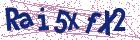# A robust method for optimal control problems governed by system of Fredholm integral equations in mechanics

Document Type : Research Article

Author

Department of Educational Mathematics, Farhangian university, Tehran, Iran.

Abstract

This essay considers an optimal control problem (OCP) governed by a system of Fredholm integral equations (FIE). In this paper, collocation approach with utilizing Lagrange polynomials is introduced to transform the OCP into a nonlinear programming problem (NLP). An eﬀicient op-timization method in Mathematica software is utilized to solve NLP. The convergence analysis is discussed, which show the theoretical structure behind the propounded technique under some assumptions. In this es-say, computational outcomes are given to demonstrate the adaptability, forthrightness, and relationship of the calculations manufactured. A prac-tical real-world problem involving hanging chain in classical mechanic is also dissolved utilizing the approach proposed.

Keywords20.1001.1.24236977.2023.13.2.6.9

Main Subjects

#### References

 Abu Arqub, O. and Shawagfeh, N. Solving optimal control problems of Fredholm constraint optimality via the reproducing kernel Hilbert space method with error estimates and convergence analysis, Math. Methods Appl. Sci. 44(10) (2021), 7915–7932.
 Alipour, M. and Soradi-Zeid, S. Optimal control of time delay Fredholm integro-differential equations, J. Math. Model. 9(2) (2021), 277–291.
 Almasieh, H. and Roodaki, M. Triangular functions method for the so-lution of Fredholm integral equations system, Ain Shams Eng. J. 3(4)(2012), 411–416.
 Babolian, E., Biazar, J. and Vahidi, A.R. The decomposition method applied to systems of Fredholm integral equations of the second kind, Appl. Math. Comput. 148(2) (2004), 443–452.
 Baghani, O. Second Chebyshev wavelets (SCWs) method for solving finite-time fractional linear quadratic optimal control problems, Math. Comput. Simul. 190 (2021), 343–361.
 Baghani, O. SCW-iterative-computational method for solving a wide class of nonlinear fractional optimal control problems with Caputo deriva-tives, Math. Comput. Simul. 202 (2022), 540–558.
 Basit, M. and Khan, F. An effective approach to solving the system of Fredholm integral equations based on Bernstein polynomial on any finite interval, Alex. Eng. J. 61(4) (2022), 2611–2623.
 Borzabadi, A.H., Fard, O.S. and Mehne, H.H. A hybrid algorithm for approximate optimal control of nonlinear Fredholm integral equations, Int. J. Math. 89(16) (2012), 2259–2273.
 Canuto, C., Hussaini, M.Y., Quarteroni, A. and Zang, T.A. Spectral methods in fluid dynamics, Springer Verlag, New York, 1988.
 Ebrahimzadeh, A., Khanduzi, R., Panjeh Ali Beik, S. and Baleanu, D. Research on a collocation approach and three metaheuristic techniques based on MVO, MFO, and WOA for optimal control of fractional differ-ential equation, J. Vib. Control (2021), 10.1177/10775463211051447.
 Ebrahimzadeh, A. and Panjeh Ali Beik, S. Application of spectral meth-ods to solve nonlinear buckling analysis of an elastic beam, Casp. J. Math. Sci., (CJMS) peer 10(1) (2021), 68–76.
 Habibli, M., Noori Skandari, M.H. Fractional Chebyshev pseudospectral method for fractional optimal control problems, Optim Control Appl Meth. 40(3) (2019), 558–572.
 Heydari, M.H., Razzaghi, M. and Avazzadeh, Z. Orthonormal piecewise Bernoulli functions: Application for optimal control problems generated using fractional integro-differential equations, J. Vib. Control (2022), 10775463211059364.
 Jerri, A.J. Introduction to Integral Equations with Applications,Wiley-Interscience, NewYork, 1999.
 Kaneko, H. and Xu, Y. Numerical solutions for weakly singular Fredholm integral equations of the second kind, Applied Numerical Mathematics 7 (1991), 167–177.
 Keyanpour, M. and Akbarian, T. Optimal control of Fredholm integral equations, Int. J. Applied Math. and Information Sci 5(3) (2011), 514–524.
 Kreyszig, E. Introductory Functional Analysis With Applications, John Wiley, New York, 1978.
 Lefebvre, M. Optimal control of an Ornstein-Uhlenbeck process, Stochas-tic Processes and their Applications 24(1) (1987), 89–97.
 Maleknejad, K. and Ebrahimzadeh, A. Optimal control of Volterra integro-differential systems based on Legendre wavelets and collocation method, Int. J. Comput. Sci 8(7) (2014), 1040–1044.
 Maleknejad, K. and Ebrahimzadeh, A. The use of rationalized Haar wavelet collocation method for solving optimal control of Volterra integral equations, J. Vib. Control 21(10) (2015), 1958–1967.
 Maleknejad, K., Aghazadeh, N. and Rabbani, M. Numerical solution of second kind Fredholm integral equations system by using a Taylor-series expansion method, Appl. Math. Comput. 175(2) (2006), 1229–1234.
 Rashidinia, J. and Zarebnia, M. Convergence of approximate solution of system of Fredholm integral equations, J. Math. Anal. Appl. 333(2) (2007), 1216–1227.
 Rivlin, T.J. An introduction to Approximation of functions, Dover, Net-work, 1969.
 Roubíček, T. Optimal control of nonlinear Fredholm integral equations, J. Optim. Theory Appl. 97(3) (1998), 707–729.
 Safaie, E., Farahi, M.H. and Farmani Ardehaie, M. An approximate method for numerically solving multi-dimensional delay fractional opti-mal control problems by Bernstein polynomials, Comput. Appl. Math. 34(3) (2015), 831–846.
 Wazwaz, A.M. Nonlinear Singular Integral Equations. In Linear and Nonlinear Integral Equations, Springer, Heidelberg, Berlin, 2011.
 Xiaobing, P., Yang, X., Skandari, M.H.N., Tohidi, E. and Shateyi, S. A new high accurate approximate approach to solve optimal control prob-lems of fractional order via eﬀicient basis functions, Alex. Eng. J. 61(8) (2022), 5805–5818.